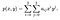# Resizing Images using Various Interpolation Techniques

Following up on my previous article on getting crisper images by manual resizing, another method used is through interpolation. Interpolation is a method of constructing new data points within the range of a discrete set of known data points. We interpolate (or estimate) the value of that function for an intermediate value of the independent variable.

There are various types of interpolation. Let’s focus on three of them —

# 1.Nearest Neighbour Interpolation

This type of interpolation is the most basic. We simply interpolate the nearest pixel to the current pixel. Assuming, we index the values of the pixels from 0. The pixels of the below 2x2 image will be as follows: {‘10’:(0,0), ‘20’: (1,0), ‘30’:(0,1), ‘40’: (1,1)}

We then project this image on the 4x4 image we require to find the pixels. We find the unknown pixels to be at (-0.5, -0.5), (-0.5, 0.5) and so on…

Now compare the values of the known pixels to the values of the nearest unknown pixels. Thereafter, assign the nearest value i.e P(-0.5,-0.5) as 10 which is the value of the pixel at (0,0).

The result is as follows -

As we can see, we get a 4x4 image where every pixel looks bigger as compared to the original image. Since this method simply looks for the nearest neighbour and computes one pixel at a time, it takes the least processing time.

To use this type of interpolation to resize an image in openCV we use the resize function with the cv2.INTER_NEAREST interpolation flag.

`import numpy as npimport cv2from matplotlib import pyplot as pltimg = cv2.imread("cube.png", -1); h, w, c = img.shape;img_n = cv2.resize(img, (w//2, h//2), interpolation = cv2.INTER_NEAREST);img_n = cv2.resize(img_n, (w, h), interpolation = cv2.INTER_NEAREST);`

This snippet of code outputs the following result -

This form of interpolation simply makes each pixel bigger and is often useful when we want to resize images which do not have as complex details like a bar code.

# 2. Bilinear interpolation

In bilinear interpolation we take the values of four nearest known neighbours (2x2 neighbourhood) of unknown pixels and then take the average of these values to assign the unknown pixel.

Let’s first understand how this would work on a simple example. Suppose we take a random point say (0.75, 0.25) which is in the middle of four points -

(0,0),(0,1),(1,0),(1,1).

We first find the values at points A(0.75, 0) and B(0.75, 1) using linear interpolation. Linear interpolation is basically taking an approximation of a point between two points by scaling the point according to how close it is to the respective two points.

We then find the value of the pixel required (0.75,0.25) using linear interpolation on points A and B.

Now that we understand how the values are found, let us take it in the context of the 2x2 image that had taken for the nearest neighbour interpolation.

Consider the 2x2 image to be projected onto a 4x4 image but only the corner pixels retain the values. The remaining pixels which are technically in the middle of the four are then calculated by using a scale to assign weights depending on the closer pixel.

For example, consider pixel (0,0) to be 10 and pixel (0,3) to be 20. Pixels (0,1) will be calculated by taking (0.75 * 10) + (0.25 * 20) which gives us 12.5. Similarly on running linear interpolation on the image while resizing, the effect will be as follows:

Bilinear interpolation has a longer processing time than nearest neighbour interpolation as it takes in the value of four pixels to compute the interpolated pixel. However, it gives a smoother output.

To use this type of interpolation to resize an image in openCV we use the resize function with the cv2.INTER_LINEAR interpolation flag. Import the same libraries as given under the nearest neighbour interpolation method above, read the image using cv2 and then resize with the cv2.INTER_LINEAR interpolation flag.

`img_b = cv2.resize(img, (w//2, h//2), interpolation = cv2.INTER_LINEAR);img_b = cv2.resize(img_b, (w, h), interpolation = cv2.INTER_LINEAR);`

# 3. Bicubic Interpolation

In bicubic interpolation we take 16 pixels around the pixel to be interpolated (4x4 neighbourhood) as compared to the 4 pixels (2x2 neighbourhood) we take into account for bilinear interpolation.

Considering a 4x4 surface, we can find the values of the interpolated pixels using this formula:Source(Wikipedia)

The interpolation problem consists of determining the 16 coefficients aᵢⱼ . These coefficients can be determined from the p(x, y) values which are attained from the matrix of pixels and partial derivatives of individual pixels.

Upon calculating the coefficients, we then multiply them with the weights of the known pixels and interpolate the unknown pixels. Let us take the same input 2x2 image we took in the two examples above. Upon bicubic interpolation, we get the following result:

Now, in order to execute this interpolation using cv2, we will once agin call the resize function but this time with the cv2.INTER_CUBIC interpolation flag. Once again, run the code after importing all the necessary libraries and reading the image using

`img_c = cv2.resize(img, (w//2, h//2), interpolation = cv2.INTER_CUBIC);img_c = cv2.resize(img_b, (w, h), interpolation = cv2.INTER_CUBIC);`

This produces noticeably sharper images than the previous two methods and balances processing time and output quality. It is standard. in many editing programs, printer drivers and in-camera interpolation.

Thus, we can see that different interpolation techniques have different use cases. Hence, it is important to understand the type of interpolation that will be most useful when resizing images.

Happy coding to you!

Data Science and Machine Learning Enthusiast

## More from Annmay Sharma

Data Science and Machine Learning Enthusiast